# Solution assignment 08 Tangent line to graph

### Assignment 8

The line: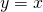intersects the parabola: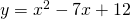in two points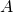en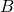.
The tangent lines at these points intersect in a point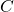.
Calculate the coordinates of.

### Solution

First we calculate the intersection points, thus we have to solve the following equation: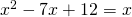We rewrite this:and we can solve this equation by factorizing: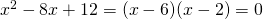and thus: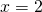orThe corresponding intersection points are: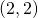and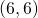In order to determine the slopes of the tangent line(s) at these points we have to differentiate the function of the parabola:For the slope of the tangent line atwe find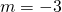and for the one atwe find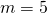. The equation of one tangent line is: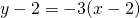or: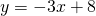The equation of the other tangent line is: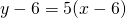or: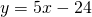We find the intersection point of these tangent lines by solving the equation:The solution of the equation is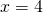and the corresponding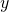-value is. The pointhas the coordinates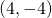.